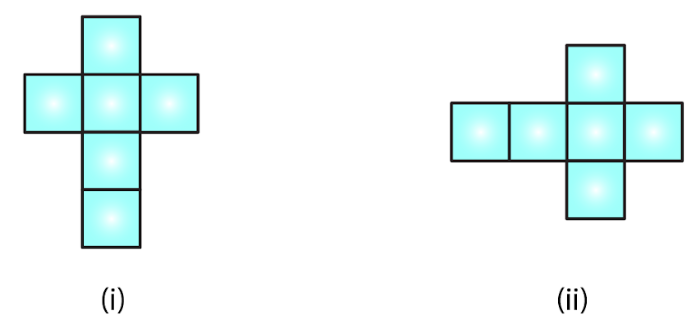# RD Sharma Solutions for Class 7 Maths Chapter 19 Visualising Solid Shapes

The PDF of RD Sharma Solutions for Class 7 Maths Chapter 19 Visualising Solid Shapes are downloaded from the given links. Class 7 is a stage where several new topics are introduced. Our experts formulate RD Sharma Solutions to help students in their exam preparation to achieve excellent marks in Maths.  Let us have a look at some important topics being discussed in this chapter.

• One-dimensional figures
• Two-dimensional figures
• Three-dimensional figure
• Faces, Edges and vertices of
• Cube
• Cuboid
• Pyramid
• Cylinder
• Cone
• sphere
• Nets for building 3D – Shapes
• Euler’s formula

RD Sharma Solutions for Class 7 help students to get a good score in the examinations. It also provides extensive knowledge about the subject, as Class 7 builds a foundation in their academic career. The solutions are stepwise and detailed to make learning easy for students.

## Download the PDF of RD Sharma Solutions For Class 7 Maths Chapter 19 Visualising Solid Shapes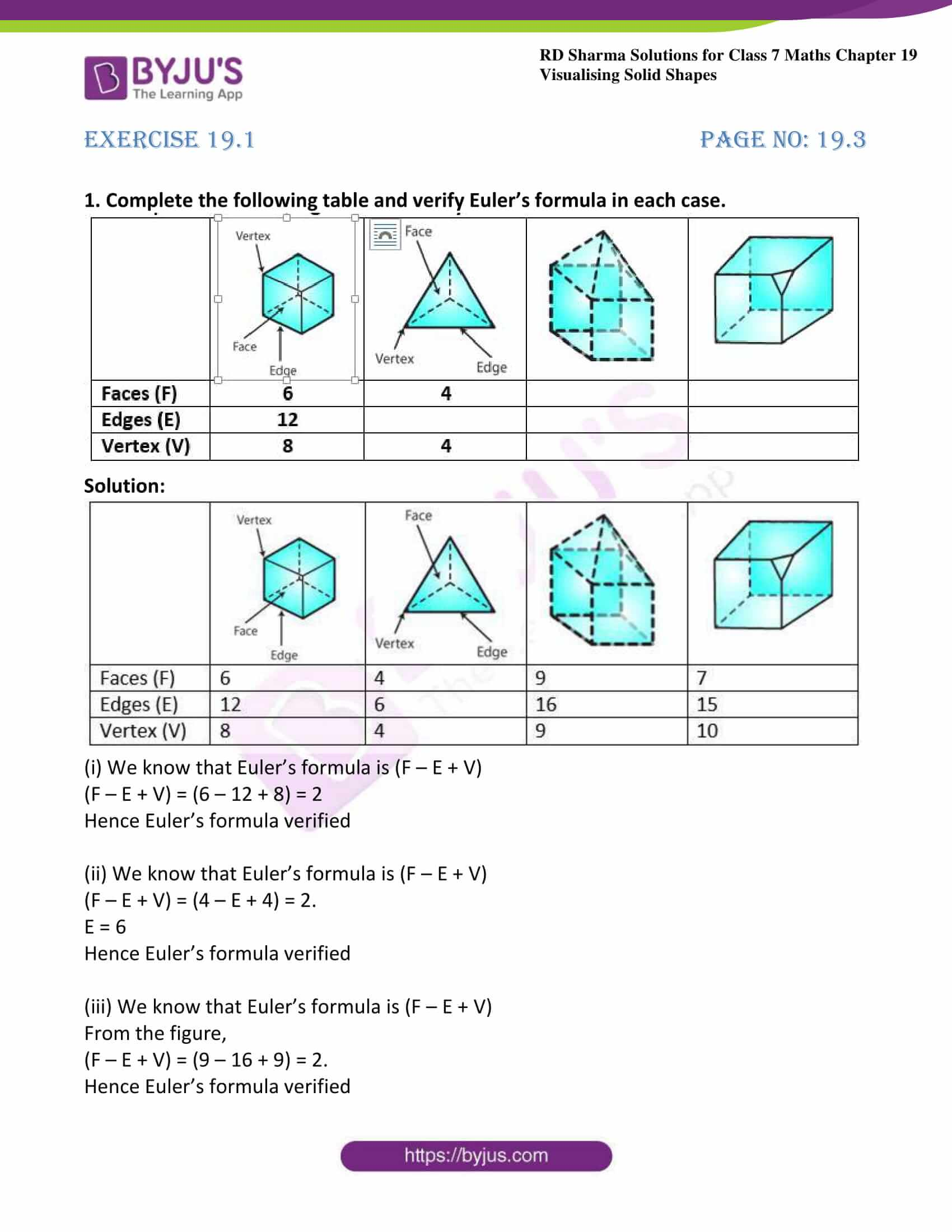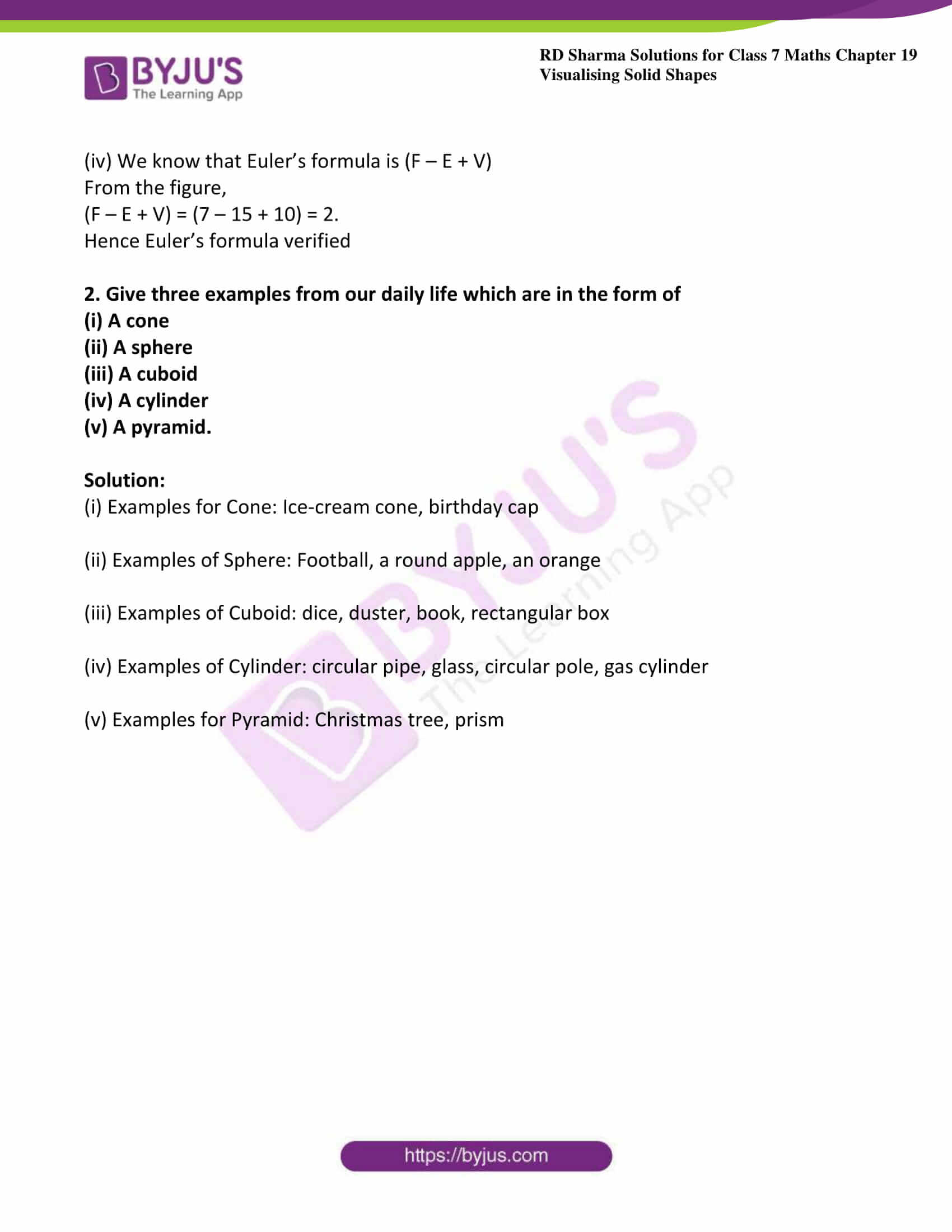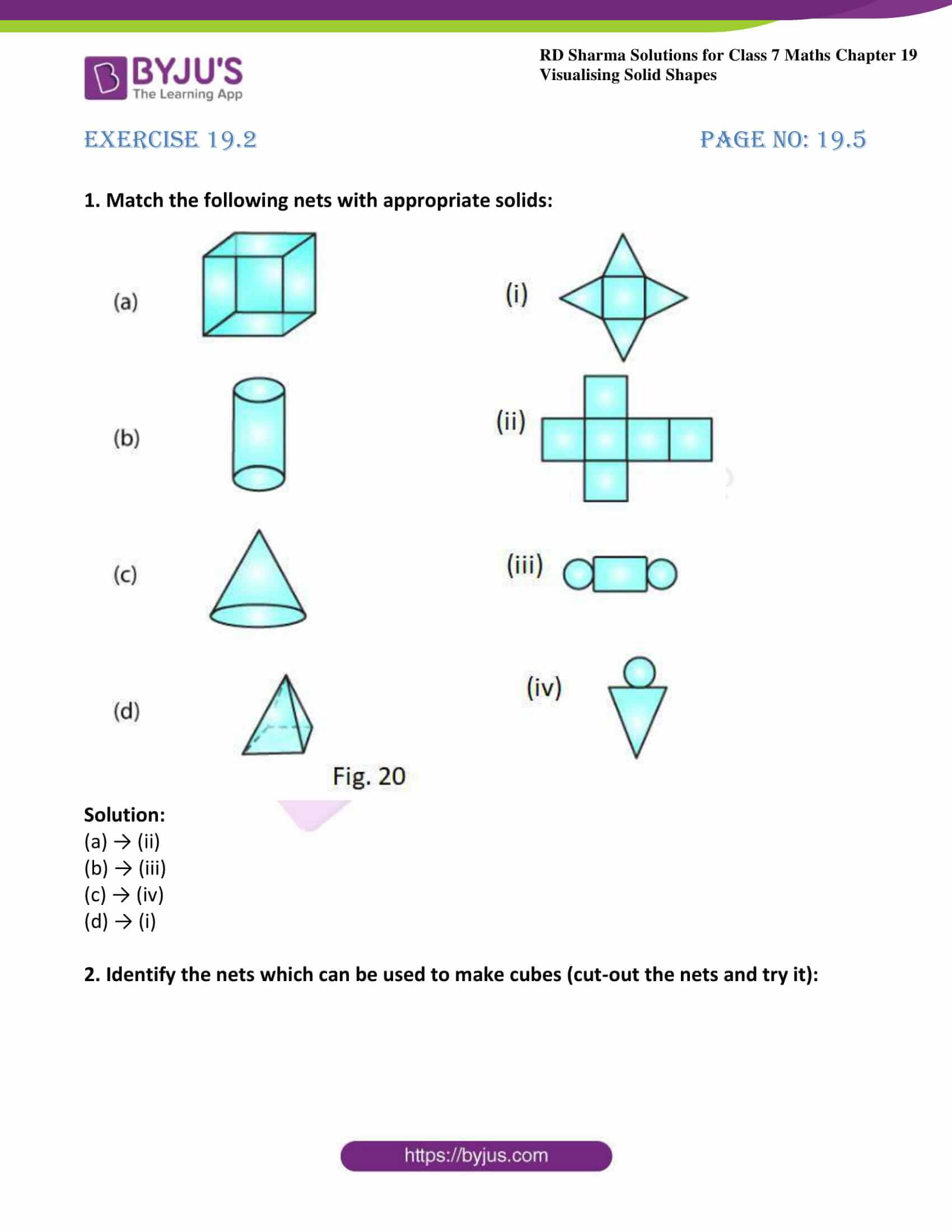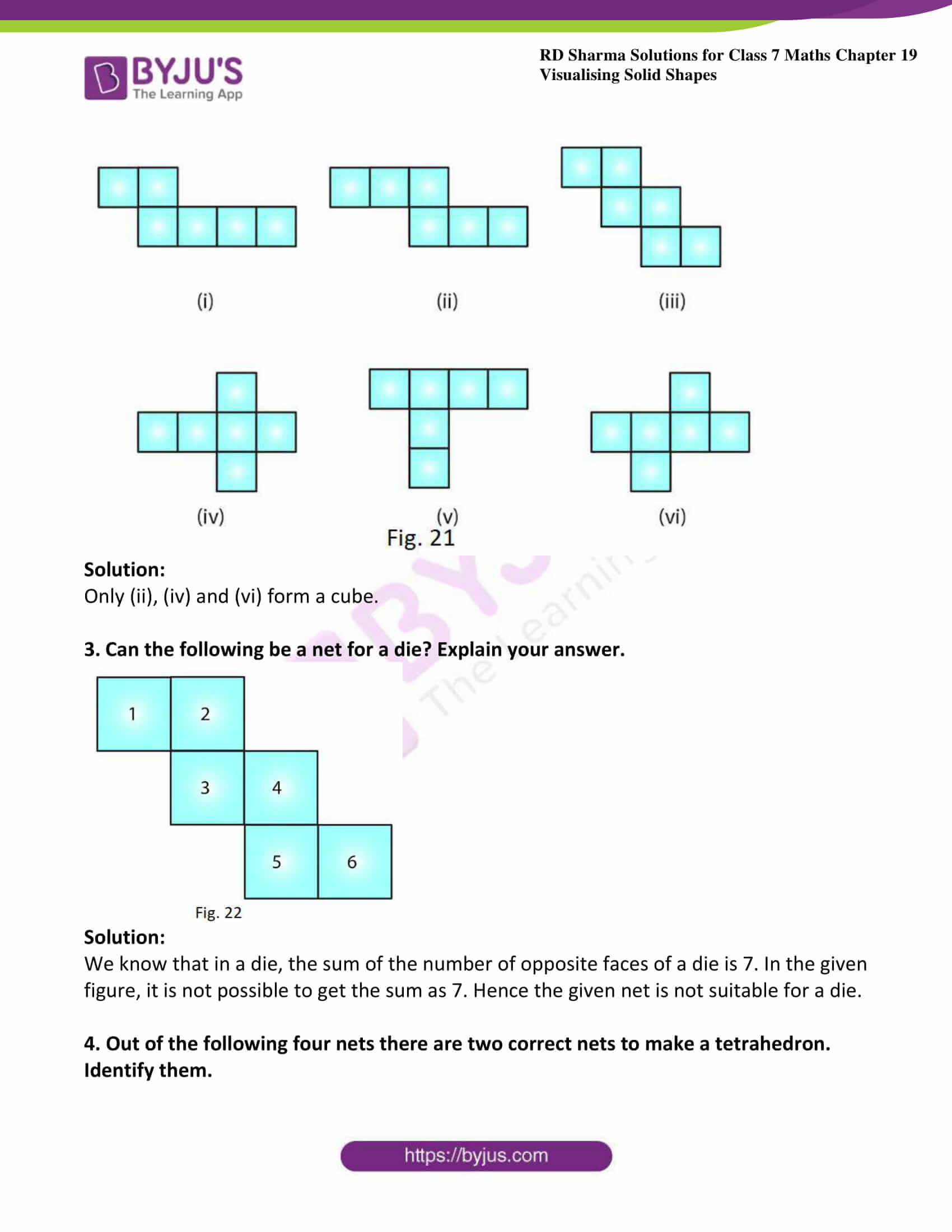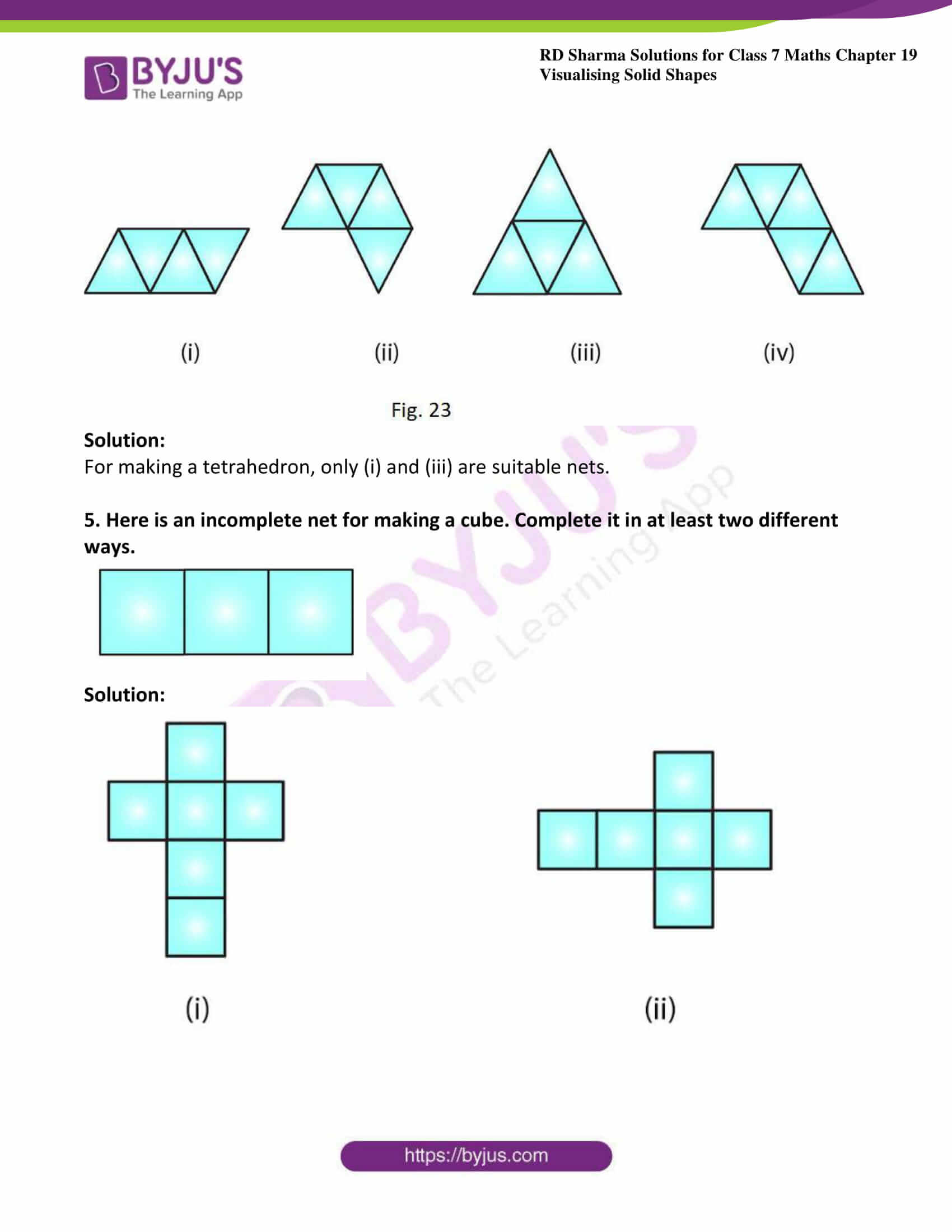### Access answers to Maths RD Sharma Solutions For Class 7 Chapter 19 – Visualising Solid Shapes

Exercise 19.1 Page No: 19.3

1. Complete the following table and verify Euler’s formula in each case.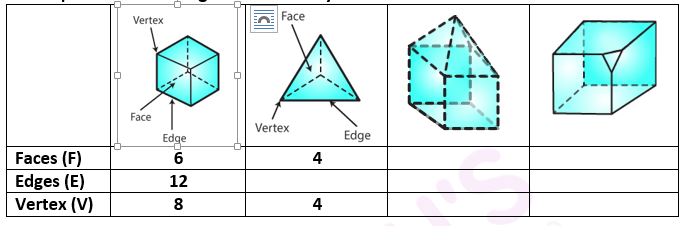Solution: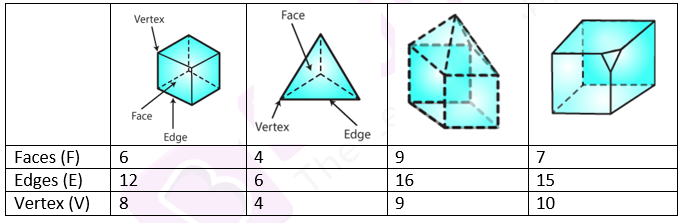(i) We know that Euler’s formula is (F – E + V)

(F – E + V) = (6 – 12 + 8) = 2

Hence Euler’s formula verified

(ii) We know that Euler’s formula is (F – E + V)

(F – E + V) = (4 – E + 4) = 2.

E = 6

Hence Euler’s formula verified

(iii) We know that Euler’s formula is (F – E + V)

From the figure,

(F – E + V) = (9 – 16 + 9) = 2.

Hence Euler’s formula verified

(iv) We know that Euler’s formula is (F – E + V)

From the figure,

(F – E + V) = (7 – 15 + 10) = 2.

Hence Euler’s formula verified

2. Give three examples from our daily life which are in the form of

(i) A cone

(ii) A sphere

(iii) A cuboid

(iv) A cylinder

(v) A pyramid.

Solution:

(i) Examples for Cone: Ice-cream cone, birthday cap

(ii) Examples of Sphere: Football, a round apple, an orange

(iii) Examples of Cuboid: dice, duster, book, rectangular box

(iv) Examples of Cylinder: circular pipe, glass, circular pole, gas cylinder

(v) Examples for Pyramid: Christmas tree, prism

Exercise 19.2 Page No: 19.5

1. Match the following nets with appropriate solids: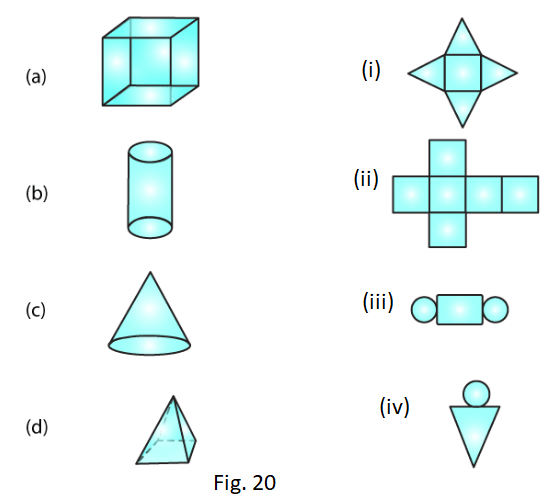Solution:

(a) → (ii)

(b) → (iii)

(c) → (iv)

(d) → (i)

2. Identify the nets which can be used to make cubes (cut-out the nets and try it):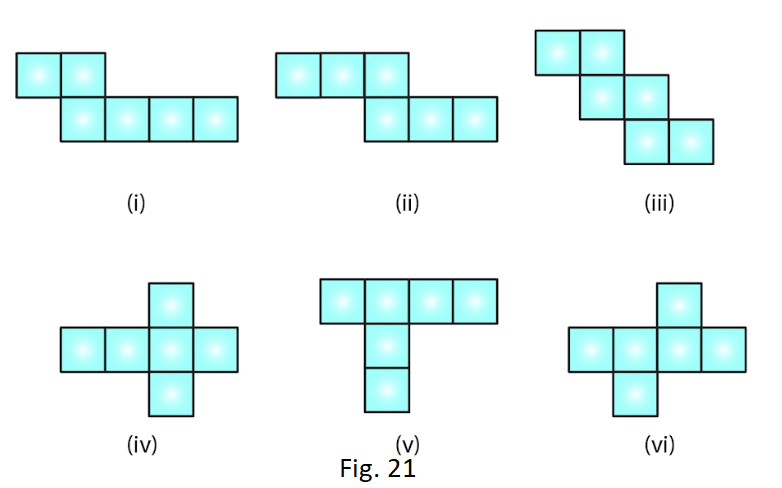Solution:

Only (ii), (iv) and (vi) form a cube.

3. Can the following be a net for a die? Explain your answer.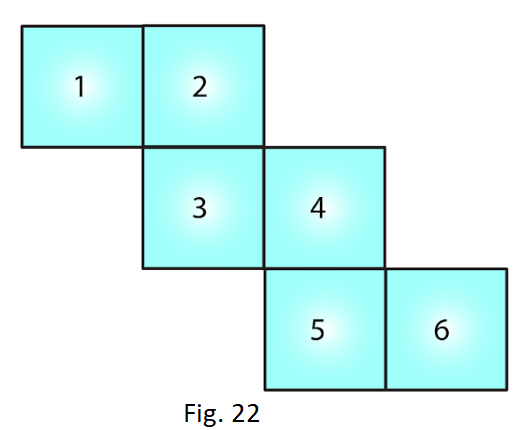Solution:

We know that in a die, the sum of the number of opposite faces of a die is 7. In the given figure, it is not possible to get the sum as 7. Hence the given net is not suitable for a die.

4. Out of the following four nets there are two correct nets to make a tetrahedron. Identify them.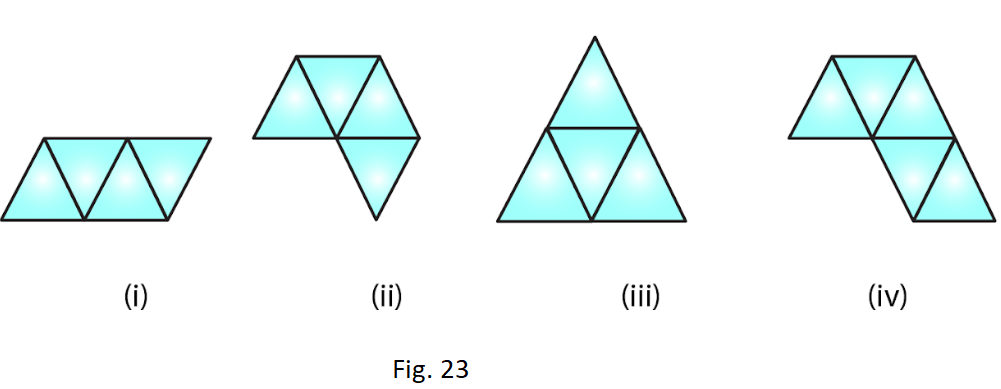Solution:

For making a tetrahedron, only (i) and (iii) are suitable nets.

5. Here is an incomplete net for making a cube. Complete it in at least two different ways.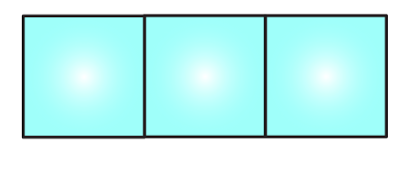Solution: